# Electrochemistry Intro Review Week of 111918 Geoff Geberth

• Slides: 17
Download presentation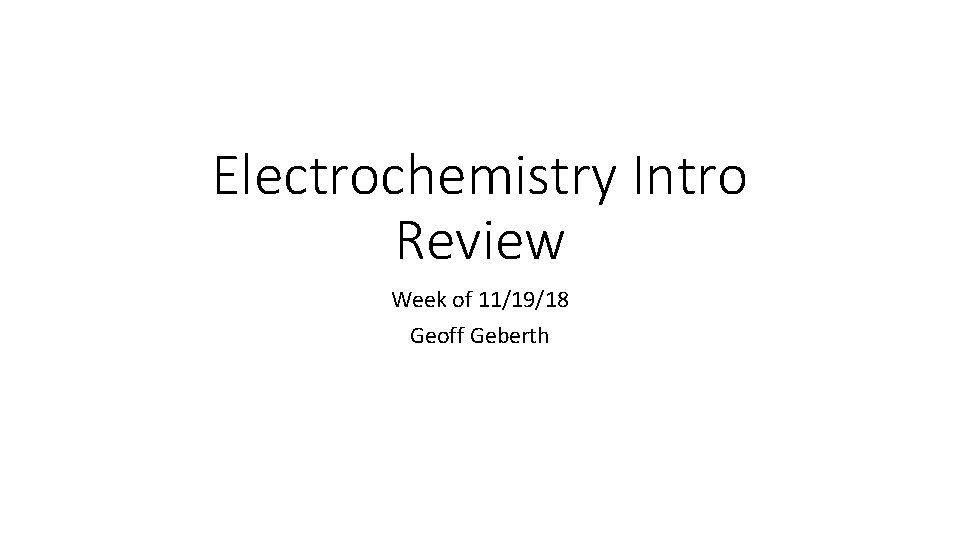Electrochemistry Intro Review Week of 11/19/18 Geoff Geberth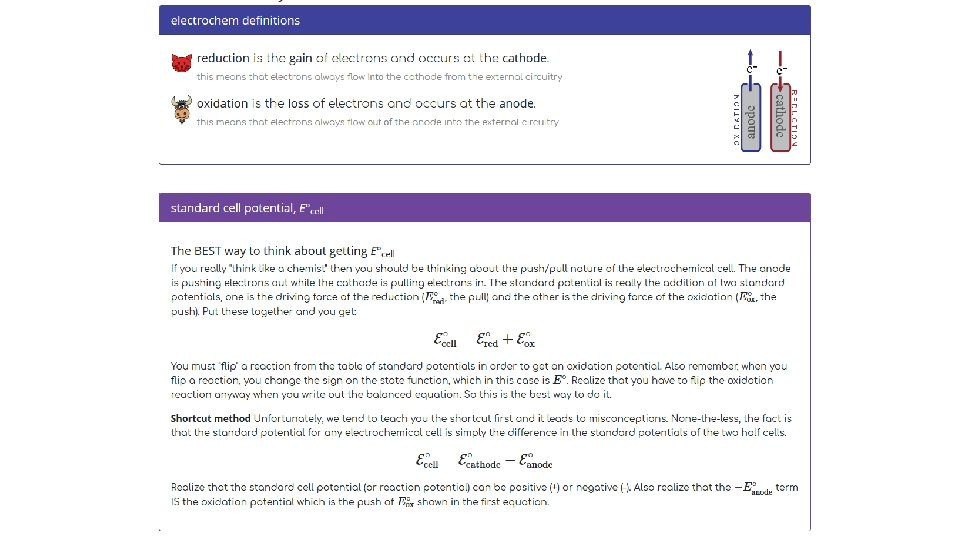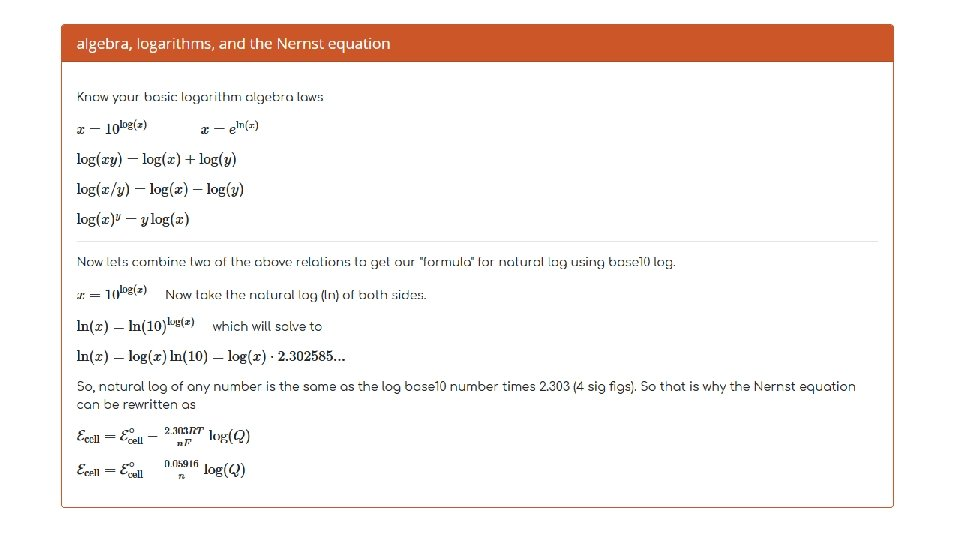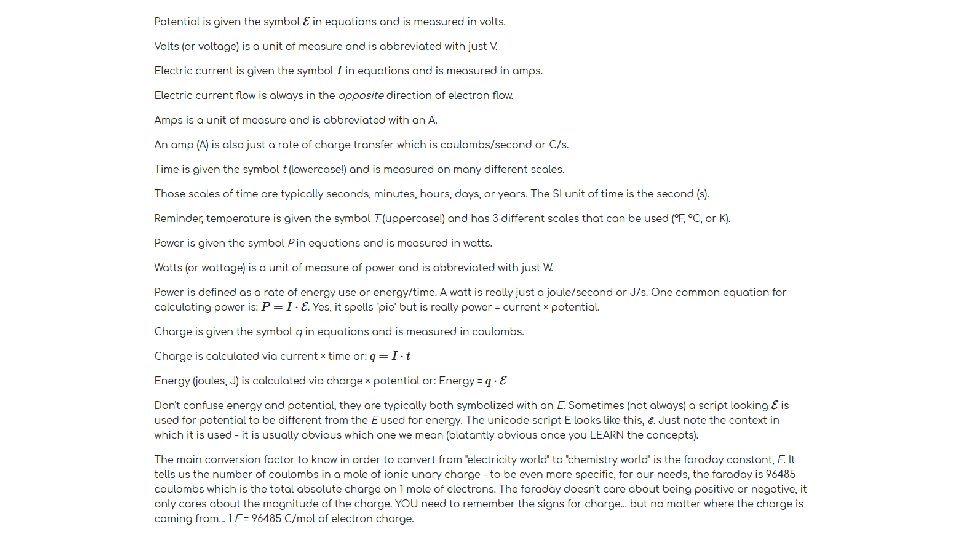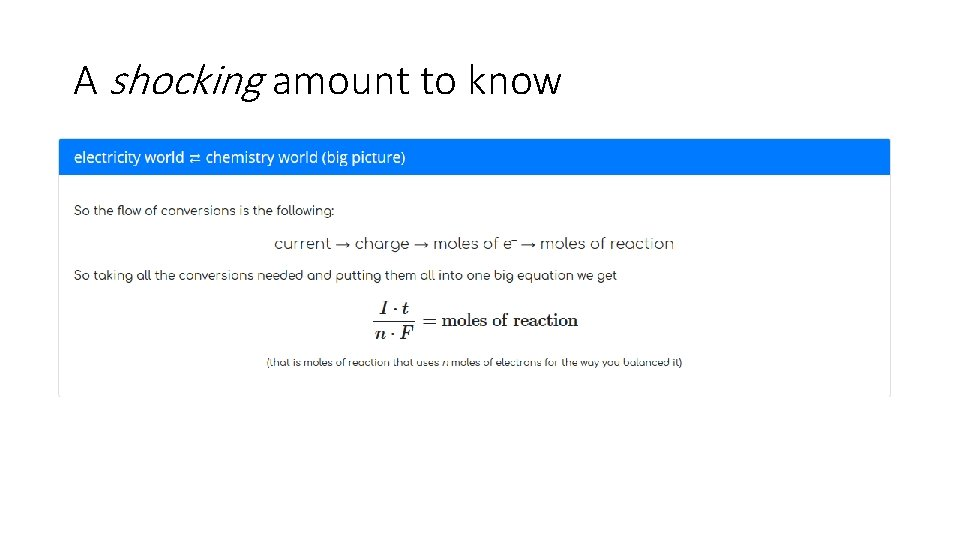A shocking amount to know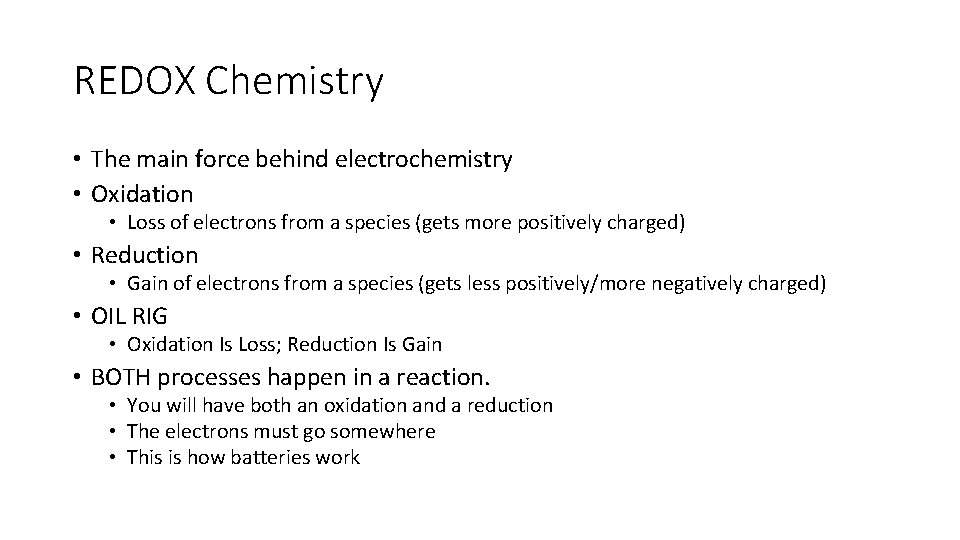REDOX Chemistry • The main force behind electrochemistry • Oxidation • Loss of electrons from a species (gets more positively charged) • Reduction • Gain of electrons from a species (gets less positively/more negatively charged) • OIL RIG • Oxidation Is Loss; Reduction Is Gain • BOTH processes happen in a reaction. • You will have both an oxidation and a reduction • The electrons must go somewhere • This is how batteries work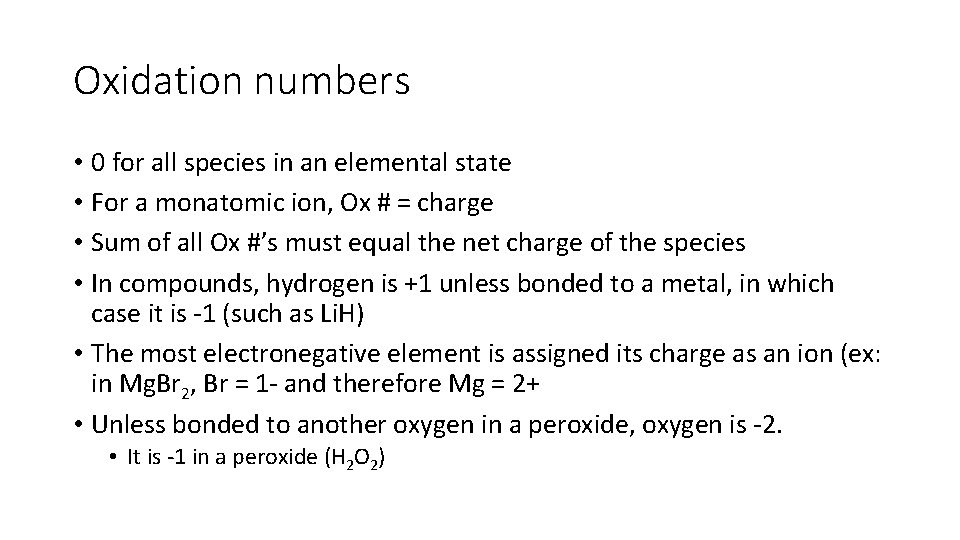Oxidation numbers • 0 for all species in an elemental state • For a monatomic ion, Ox # = charge • Sum of all Ox #’s must equal the net charge of the species • In compounds, hydrogen is +1 unless bonded to a metal, in which case it is -1 (such as Li. H) • The most electronegative element is assigned its charge as an ion (ex: in Mg. Br 2, Br = 1 - and therefore Mg = 2+ • Unless bonded to another oxygen in a peroxide, oxygen is -2. • It is -1 in a peroxide (H 2 O 2)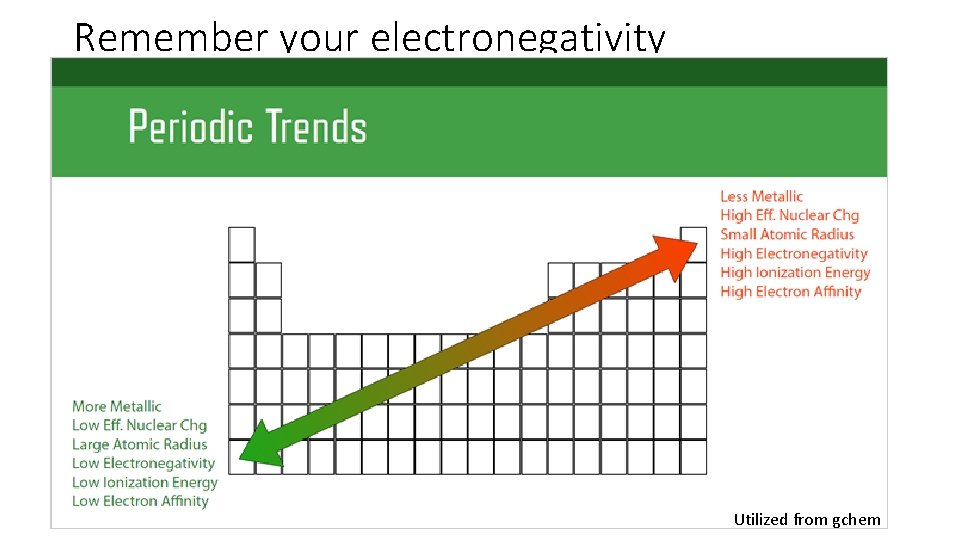Remember your electronegativity Utilized from gchemLet’s Practice! •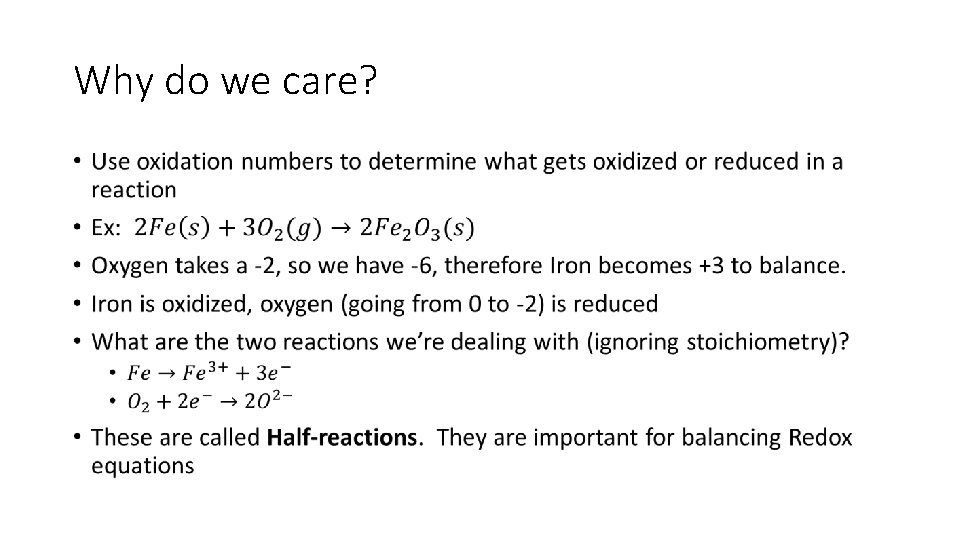Why do we care? •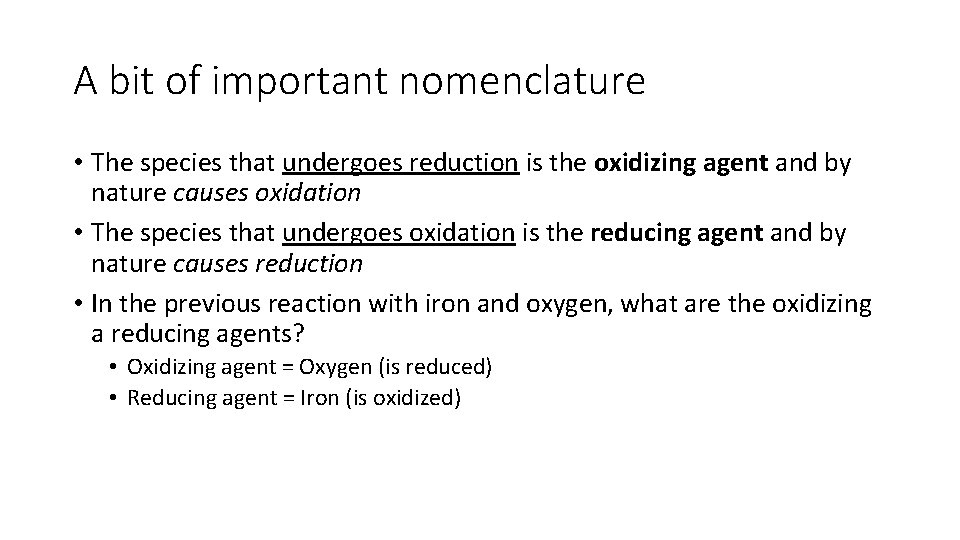A bit of important nomenclature • The species that undergoes reduction is the oxidizing agent and by nature causes oxidation • The species that undergoes oxidation is the reducing agent and by nature causes reduction • In the previous reaction with iron and oxygen, what are the oxidizing a reducing agents? • Oxidizing agent = Oxygen (is reduced) • Reducing agent = Iron (is oxidized)Balancing Redox reactions (the half-reaction method)(acidic conditions) Identify your half reactions For each half reaction, balance all elements EXCEPT hydrogen and oxygen Balance the oxygens by adding H 2 O Balance the hydrogens by adding H+ Balance the total charge using electrons (e-) Match the electron count for the two half-reactions by multiplying stoichiometric coefficients of the half-reactions • Combine the two reactions to make the overall, cancelling any species on both sides • Check your work to make sure you have the same components on both sides • • •Let’s practice • Combine your balanced half reactions! Far more importantly, lead acid batteries are what you find in standard (non-hybrid or electric) automobiles!Basic conditions • First, balance under acidic conditions • Add the same number of OH- ions to the side of the eqn with H+ so that the acid is neutralized into water • Add the same number of OH- to the other side of the reaction • On the side with both H+ and OH-, cross them out and replace with water (This is the switch from acidic to basic conditions) • Cancel any waters that appear on both sides of the equation • Double check the balance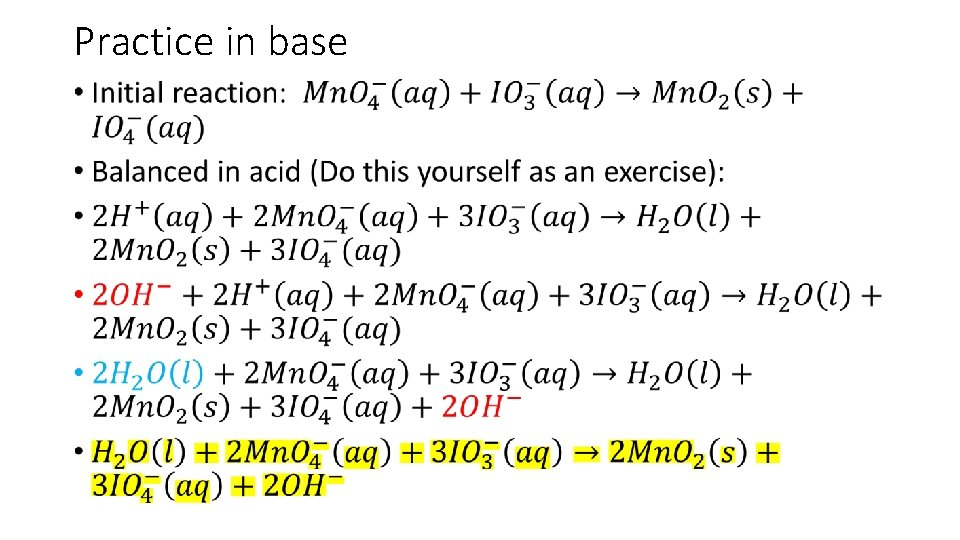Practice in base •